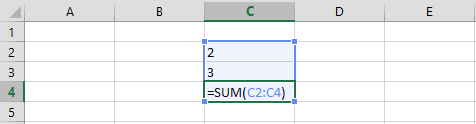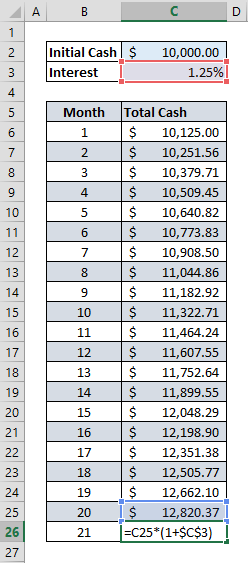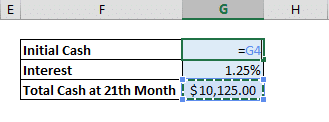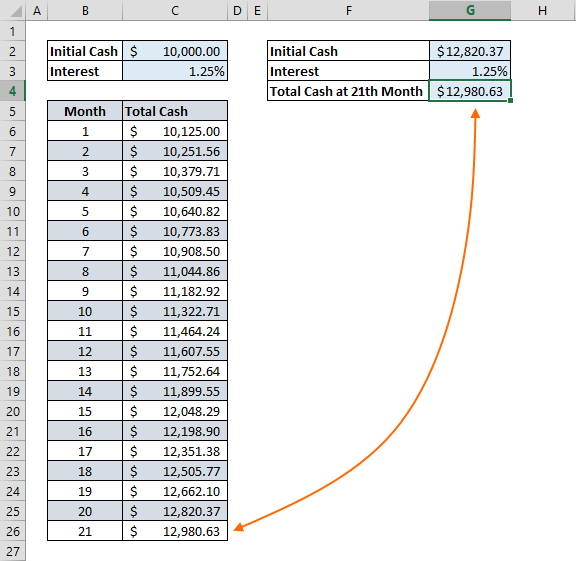In Excel, you typically type in formulas to get results and it’s pretty common to reference other cells in the process. However, what happens if you reference the cell itself, either directly or indirectly? This means an infinite loop of calculations! Luckily, Excel has solutions to deal with these kinds of situations.

Circular Reference means that your formula is trying to calculate the origin cell. Typically, this is considered an error. However, there are times where this error can actually be useful and you might to want to create a circular reference on purpose. Let’s take a closer look at this phenomenon with some examples. You can download the sample workbook here.

### Direct Reference

If you go to the cell C3, for example, and then type in “=C3”, you would confuse Excel a bit because this is cell is now pointing to itself, thus creating a circular reference. Doing the same thing inside a formula will also have the same effect (i.e. =IF(C3,…,…) or =C3+10).Another common example is including the original cell when selecting a range from adjacent cells. For example, including the cell C4 in the sum formula =SUM(C2:C4) will transform this into a circular reference.### Indirect Reference

A cell that is targeting a cell that is pointing to the originating cell will create an indirect reference. In other words, circular reference can be established by two cells targeting each other. For example, entering “=D3” into cell B3, while cell D3 contain a reference from B3 cell (“=B3” in this case).## Why is this a problem?

Excel warns the user when there’s an occurrence of a circular reference, because a circular reference essentially means endless loops. Telling Excel to repeat the same calculation over and over for an infinite number of times doesn’t make too much sense. To prevent freezing your computer, Excel simply ignores circular references.

Let’s take a look at this warning message. You’ll get the following message after typing in a circular referenced formula,Note that Excel doesn’t give the same error for additional circular formula references. On Excel’s status bar, the latest cell with a circular reference will be shown. This means that there might be more than one such reference on this tab. Here is what it looks like,Excel also keeps a list of all circular references, which can be accessed from Error Checking option on the Formula tab,## How to get rid of them

Unfortunately, there is no easy way to remove or fix unwanted circular references. You’re going to need to use one of the ways mentioned before and resolve them one by one. To find out about what’s causing the issue, you can use the Formula Auditing option under Formula tab. See our guide to learn how to do this, https://www.spreadsheetweb.com/identifying-analyzing-spreadsheets-formula-auditing/

## Can they be useful?

Yes! Although, only under some circumstances. You may intentionally want to calculate a circular formula block more than few times. Excel allows this “iterative” calculation approach using limits.

To activate and use circular references you must first activate them by checking Enable iterative calculations option under the File menu.

Go to File > Options > Formulas > Calculation options in Excel 2016, Excel 2013 and Excel 2010.In Excel 2007, you need to go to Office button > Excel options > Formulas > Iteration area

In Excel 2003 and earlier, you need to go toMenu > Tools > Options > Calculation

Enabling iterative calculations will bring up two additional inputs in the same menu,

• Maximum Iterations determines how many times Excel is to recalculate the workbook
• Maximum Change determines the maximum difference between values of iterative formulas. Note that smaller number means accurate results.

Iterative calculations stop when one of the conditions defined above are matched.

### Use Cases

#### Calculating Future Value of an Investment

Let’s assume that we have \$10,000 want to invest this money in a CD account. The monthly interest in this option is 1.25%. Download the sample workbook for this use case here. To calculate the total value at the end of 21st month, we’re going to calculate each month and add the interest to the previous month.First, enter the starting money, interest, and the basic total value function,

=value * ( 1 + interest rate)Then, select the cell with the initial cash value and add the reference of the total value function.This move will cause a circular reference warning if iterative calculations are not enabled. If you haven’t done so already, enable this option and set Maximum Iterations to 20 to find the interest at 21st month.Press OK.#### Automatic Timestamp

Circular references can also be used to add time stamp into cells. Download the sample workbook for this use case here. Let’s assume we want to add time stamps to the orders in the table below,We can use circular references to add a time stamp when a new order information is entered.

Start with adding a new column into table to place the timestamps in. Type in the formula, =IF(A2<>"",IF(I2<>"",I2,NOW()),"")

This formula will check whether the Order Number (cell A2) was entered. If there’s a value entered and the timestamp cell is empty, then the formula will return the NOW() function.Note that pressing the Enter button will give a circular reference warning if iterative calculations are not enabled. This time Maximum Iterations or Maximum Change numbers don’t mean much because we only need a single iteration, so you can leave these two inputs in their default values.Now, every time we enter a new order and create a new row, an timestamp will be automatically printed on the Timestamp column.In its essence an error, circular references can actually be useful in some cases. It’s definitely one of those features that most users haven’t even heard of. However, knowing your way around them (and with them!) can help you build more sophisticated Excel models.